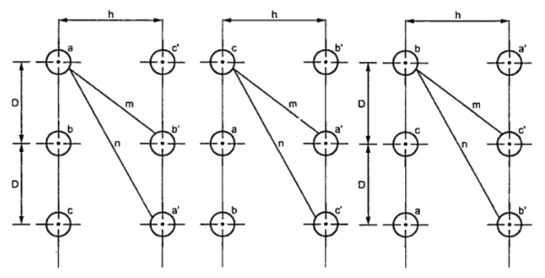### Capacitance of 3Ph Double Circuit with Unsymmetrical Spacing but Transposed

Consider the arrangement of conductors shown in the Fig. 1. It consists of three phase double circuit. The radius of each conductor r.Fig. 1

The voltage between phases a and b can be calculated in order to calculate capacitance.
One complete cycle of transposition is shown in the Fig. 1.
Similarly,
We have,  Vab + Vac = 3 Van
Also, qa + qb + qc = 0

The capacitance per phase will be 2 Can .